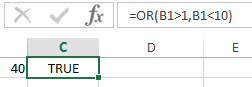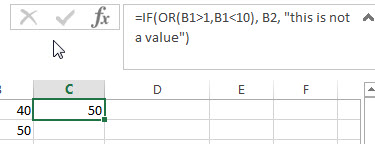# Excel OR Function

This post will guide you how to use Excel OR function with syntax and examples in Microsoft excel.

### Description

The Excel OR function used to test multiple conditions and returns TRUE if any of the conditions are TRUE. Otherwise, it will return FALSE. You can use the OR function as the logical test inside the IF function and it also can be combined with the AND function to create a new Excel Formula.

The OR function is a build-in function in Microsoft Excel and it is categorized as a Logical Function.

The OR function is available in Excel 2016, Excel 2013, Excel 2010, Excel 2007, Excel 2003, Excel XP, Excel 2000, Excel 2011 for Mac.

### Syntax

The syntax of the OR function is as below:

`=OR(logical1, [logical2], ...)`

Where the OR function arguments are:

• logical1 – This is a required argument.  The first condition or logical value that you want to test.
• logical2 – This is an optional argument. The second condition or logical value that you want to test. And it can be up to 255 logical test argument in Excel 2007 or later. And it only can up to 30 arguments in excel 2003.

### Excel OR Function Examples

The below examples will show you how to use Excel OR Function to test a number of supplied conditions and return TRUE or FALSE.

1# To return TRUE if B1 is greater than 1 or less than 10, otherwise returns FALSE, using the following formula:

=OR(B1>1,B1<10)2# to return the value in Cell B2 if it is greater than 1 or less 10, otherwise returns the message “this is not a value”, using the following formula:

=IF(OR(B1>1,B1<10), B2, “this is not a value”)Related Functions

• Excel AND function
The Excel AND function returns TRUE if all of arguments are TRUE, and it returns FALSE if any of arguments are FALSE.The syntax of the AND function is as below:= AND (condition1,[condition2],…) …
• Excel IF function
The Excel IF function perform a logical test to return one value if the condition is TRUE and return another value if the condition is FALSE….

### Related Formulas

• Excel IF formula with NOT logical function
If you want to check several test conditions in an excel formula,then take different actions. You can use NOT function in combination with the AND or OR logical function in excel IF function….
• Excel IF formula with OR logical function
If you want to check if one of several conditions is met in your excel workbook, if the test is TRUE, then you can take certain action. You can use the IF function combining with OR function to construct a condition statement…
• Excel IF formula with AND & OR logical functions
If you want to test the result of cells based on several sets of multiple test conditions, you can use the IF function with the AND and OR functions at a time…